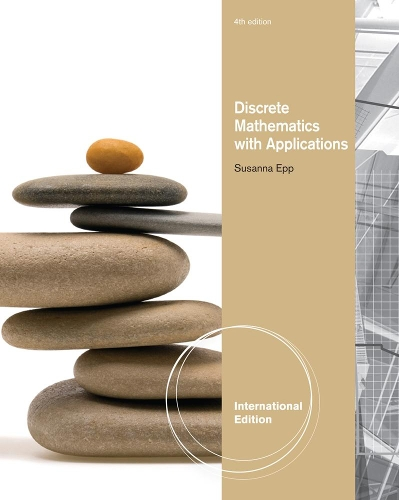•# Discrete Mathematics with Applications, International Edition (Paperback)

(author)
£78.99
Paperback 984 Pages / Published: 27/05/2011
• We can order this from the publisher

Usually dispatched within 10 working days

Susanna Epp's DISCRETE MATHEMATICS WITH APPLICATIONS, 4e, International Edition provides a clear introduction to discrete mathematics. Renowned for her lucid, accessible prose, Epp explains complex, abstract concepts with clarity and precision. This book presents not only the major themes of discrete mathematics, but also the reasoning that underlies mathematical thought. Students develop the ability to think abstractly as they study the ideas of logic and proof. While learning about such concepts as logic circuits and computer addition, algorithm analysis, recursive thinking, computability, automata, cryptography, and combinatorics, students discover that the ideas of discrete mathematics underlie and are essential to the science and technology of the computer age. Overall, Epp's emphasis on reasoning provides students with a strong foundation for computer science and upper-level mathematics courses.

Publisher: Cengage Learning, Inc
ISBN: 9780495826163
Number of pages: 984
Weight: 1700 g
Dimensions: 255 x 206 x 35 mm
Edition: 4th edition

MEDIA REVIEWS
1. SPEAKING MATHEMATICALLY. Variables. The Language of Sets. The Language of Relations and Functions. 2. THE LOGIC OF COMPOUND STATEMENTS. Logical Form and Logical Equivalence. Conditional Statements. Valid and Invalid Arguments. Application: Digital Logic Circuits. Application: Number Systems and Circuits for Addition. 3. THE LOGIC OF QUANTIFIED STATEMENTS. Predicates and Quantified Statements I. Predicates and Quantified Statements II. Statements with Multiple Quantifiers. Arguments with Quantified Statements. 4. ELEMENTARY NUMBER THEORY AND METHODS OF PROOF. Direct Proof and Counterexample I: Introduction. Direct Proof and Counterexample II: Rational Numbers. Direct Proof and Counterexample III: Divisibility. Direct Proof and Counterexample IV: Division into Cases and the Quotient-Remainder Theorem. Direct Proof and Counterexample V: Floor and Ceiling. Indirect Argument: Contradiction and Contraposition. Indirect Argument: Two Classical Theorems. Application: Algorithms. 5. SEQUENCES, MATHEMATICAL INDUCTION, AND RECURSION. Sequences. Mathematical Induction I. Mathematical Induction II. Strong Mathematical Induction and the Well-Ordering Principle. Application: Correctness of Algorithms. Defining Sequences Recursively. Solving Recurrence Relations by Iteration. Second-Order Linear Homogeneous Recurrence Relations with Constant Coefficients. General Recursive Definitions and Structural Induction. 6. SET THEORY. Set Theory: Definitions and the Element Method of Proof. Set Identities. Disproofs and Algebraic Proofs. Boolean Algebras, Russell's Paradox, and the Halting Problem. 7. PROPERTIES OF FUNCTIONS. Functions Defined on General Sets. One-to-one, Onto, Inverse Functions. Composition of Functions. Cardinality, Sizes of Infinity, and Applications to Computability. 8. PROPERTIES OF RELATIONS. Relations on Sets (add material about relational databases). Reflexivity, Symmetry, and Transitivity. Equivalence Relations. Modular Arithmetic with Applications to Cryptography. Partial Order Relations. 9. COUNTING. Counting and Probability. The Multiplication Rule. Counting Elements of Disjoint Sets: The Addition Rule. The Pigeonhole Principle. Counting Subsets of a Set: Combinations. r-Combinations with Repetition Allowed. Pascal's Formula and the Binomial Theorem. Probability Axioms and Expected Value. Conditional Probability, Bayes' Formula, and Independent Events. 10. GRAPHS AND TREES. Graphs: An Introduction. Trails, Paths, and Circuits. Matrix Representations of Graphs. Isomorphisms of Graphs. Trees: Examples and Basic Properties. Rooted Trees. Spanning Trees and a Shortest Path Algorithm. 11. ANALYZING ALGORITHM EFFICIENCY. Real-Valued Functions of a Real Variable and -Notations. Application: Efficiency of Algorithms I.?-, and ?Their Graphs. O-, Exponential and Logarithmic Functions: Graphs and Orders. Application: Efficiency of Algorithms II. 12. REGULAR EXPRESSIONS AND FINITE STATE AUTOMATA. Formal Languages and Regular Expressions. Finite-State Automata. Simplifying Finite-State Automata.